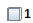Level Curves and Cross Sections - Maple Help

Home : Support : Online Help : Math Apps : Calculus : Multivariate : Level Curves and Cross Sections

Level Curves and Cross Sections

 Main Concept A level curve of the surface  is a two-dimensional curve with the equation , where k is a constant in the range of f. A level curve can be described as the intersection of the horizontal plane  with the surface defined by f. Level curves are also known as contour lines. A vertical cross section (parallel to a coordinate plane) of a surface  is a two-dimensional curve with either the equation  or the equation , where c and d are constants. Such a cross section can be described as the intersection of a vertical plane  or  with the surface defined by f. Both level curves and cross sections are helpful for visualizing and plotting multivariate functions.

Select a function from the drop-down menu or type your own function in the text box below and click "Enter" to plot it. Click the radio buttons to view either a level curve or a cross section. Use the slider to change the value of the related constant $k$, $c$, or $d$.  Click "Reset" to reset both plots.z = f(x, y) = Enter Functionx^2 + y^2 - 5x^2 - y^2sqrt(100 - x^2)-2*sqrt(x^2 + y^2) + 8x - x^3/12 - y^2/4cos(x) - sin(y)2*sin(x) + cos(y)ln(abs(x)) + yexp(x) - exp(y)cos(x)sin(cos(x*y))sin(x*cos(y))exp(sin(x + y))=More MathApps